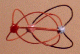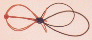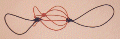# Neutron 1: 2nd Degree (N1-22S)The Neutron Shell of 1 is an un-filled shell built from two hadron strings. The equatorial string is in red. The sinusoidal string goes twice around the particle to close on itself, it is the orange string. The Pinned Vector Boson (PVB) is a lepton string pinned to two of the neutron's three Up quarks to emulate the properties of Down quarks. The lepton string is shown in black, it contributes two snap points and a negative charge current. There is no restriction that I know of which prohibits the pinning of a vector boson to two sites on the nuclear surface, so I have shown it thus, however this is an extremely young science and I could be wrong, so I also show the neutron with two pinned vector bosons. Note that the core geometry of the neutron is identical to the core geometry of the proton, differing only in the number of pinned vector bosons. The model pictured was made with a 7.5" and a 18" length of wire. All the models with sinusoidal strings have been recalculated to show the maximum length of the sinusoidal string.There should be a symmetrical particle in lepton space, a lepton form for the neutron. In the case of a lepton form, the core geometry would be composed of lepton string, whereas the pinned string would be a hadron string. It would be a neutral lepton with a mass in excess of the muon, and a very short lifetime. If it pinned a lepton string in place of the hadron string, it would have nearly the mass of an electron and it would be very hard to detect. Assuming the more massive, short-lived variety which pins a hadron string, I believe the decay mode would likely be the same as for the neutron, the decay products ultimately leading to the production of a proton and an electron.

## Stereograms of Proton (Upper Right) and Neutron ShellThe lower left and lower right shells represent the neutron, on the left the neutron is shown with two seperate P.V.B.'s, and on the right it is shown with a single doubly-pinned P.V.B.. Note that the core geometry of the proton and neutron are the same, they vary only in the number of pinning sites.

 Required Snap Points: 11 Available Snap Points: 9 Pinned Vector Bosons: 2 *L1 = 14.17424118" (Equatorial) *L2 = 36.82575882" (Sinusoidal) Model Diameter: 4.511801096" *Loop Ratio (1:2): 0.384900179 *(See Sinusoidal Strings.) Loop Equation: 1(L1) + 1(L2) = 51" Snap Point Equation: 1(3) + 1(6) = 9 A.S.P. PVB's:(+ 2 P.V.B. = 11 R.S.P.)

Copyright 1997 by Arnold J. Barzydlo
Go Back